# Regular ring (in the sense of von Neumann)

Jump to: navigation, search

An associative ring(usually with unit element) in which the equation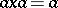is solvable for any(cf. Associative rings and algebras). The following properties are equivalent: a)is a regular ring; b) every principal left ideal ofis generated by an idempotent; c) the principal left ideals ofform a sublattice in the lattice of all left ideals which is a complemented modular lattice; d) every principal left ideal ofhas a complement in the lattice of all left ideals; e) all left-modules are flat; and f) the right analogues of the properties b)–e) hold (see , , , , ). In view of e), regular rings are sometimes called absolutely flat. A commutative ring is regular if and only if all simple modules over it are injective (see ). Every finitely-generated left (right) ideal of a regular ring is principal, and is thus distinguished as a direct summand. Every non-divisor of zero in a regular ring is invertible. The Jacobson radical of a regular ring is equal to zero. A ring of matrices over a regular ring is again a regular ring. The class of regular rings is closed under the formation of direct products and quotient rings. An ideal in a regular ring is a regular ring (possibly without unit element). If a regular ring is Noetherian or perfect (left or right), then it is a classical semi-simple ring. Every classical semi-simple ring is regular. Moreover, the endomorphism ring of a vector space over a skew-field is regular (even in the infinite-dimensional case), and so is the quotient ring of the endomorphism ring of any injective left (right) module over any ring by its Jacobson radical (see ). In particular, any left (right) self-injective ring with zero Jacobson radical is regular. The group ring of a groupover a regular ring is regular if and only if every finitely-generated subgroup ofis finite and the order of every such subgroup is invertible in the original regular ring (see ). The endomorphism rings of all free left-modules are regular only in the case whenis classically semi-simple . Countably-generated one-sided ideals of regular rings are projective .

Ifis a regular ring, then the finitely-generated submodules of the left-module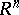of-tuples overform a complemented modular latticewhich is a sublattice of the lattice of all submodules of the module. The latticecontains a homogeneous basis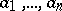, that is, these elements are independent (see Modular lattice), their sum is equal to the largest element of(namely), and every pair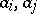is perspective, that is, they have a common complement. Conversely, every complemented modular lattice with a homogeneous basis of at least four elements is isomorphic to such a latticefor some regular ring. The latticeis isomorphic to the lattice of principal left ideals of the ring of all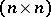-matrices over(see , ).

An important special case of a regular ring is a strictly-regular ring, in which, by definition, the equation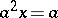is always solvable. The following properties of a regular ringare equivalent: 1)is strictly regular; 2)has no non-zero nilpotent elements; 3) all the idempotents ofare central; 4) every left (or right) ideal ofis two-sided; 5) the lattice of principal left (right) ideals ofis distributive; and 6) the multiplicative semi-group ofis an inverse semi-group (see , ).

Another subclass of the class of regular rings is that consisting of-regular rings, in which, by definition, the equationalways has an invertible element as a solution. In the class of regular rings,-regular rings are characterized by the transitivity of perspectivity in the lattice of finitely-generated submodules of the sum of two copies of the ground ring, and also by the fact that a direct sum can be contracted on a finitely-generated projective module (see , ).

A regular ring is called left continuous if a lattice of principal left ideals is a continuous geometry (cf. Orthomodular lattice). A continuous regular ring is-regular and decomposes into the direct sum of a strictly-regular ring and a self-injective ring. A pseudo-rank function can be defined on a regular ring, and it is an analogue of a measure on a Boolean algebra. It defines a pseudo-metric. The completion of a regular ring with respect to this metric turns out to be a self-injective regular ring (see ).

Regular rings are a special case of-regular rings, in which, by definition, for every elementthere are an elementand a positive integersuch that.

As two-sided analogues of regular rings, one can consider biregular rings, in which, by definition, every principal two-sided ideal is generated by a central idempotent (cf. Central algebra). Every two-sided ideal of a biregular ring is an intersection of maximal two-sided ideals. Every biregular ring with a unit is isomorphic to the ring of global sections with compact support of a sheaf of simple rings with a unit over a compact totally disconnected Hausdorff space, and any such ring of global sections is biregular (see ). In the commutative case, the classes of biregular, strictly regular and regular rings coincide, and one must substitute fields for simple rings in the last theorem above.

Baer rings are close to regular rings, and are defined by the condition that every left (or right, which is equivalent when there is a unit element) annihilator is generated by an idempotent. Examples of Baer rings are the endomorphism ring of a vector space over a skew-field and the ring of bounded operators on a Hilbert space. A Baer ring is said to be Abelian if all its idempotents are central, and (Dedekind) finite if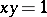implies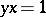. An idempotentof a Baer ringis called Abelian (finite) if the ring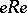is Abelian (Dedekind finite). One distinguishes the following classes of Baer rings: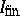, finite rings containing an Abelian idempotent that does not belong to any proper direct summand;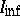, Dedekind infinite rings (that is, rings not containing non-zero finite central idempotents) containing an Abelian idempotent that does not belong to any proper direct summand;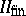(or), Dedekind finite rings without non-zero Abelian idempotents, but containing a finite idempotent that does not belong to any proper direct summand;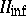, Dedekind infinite rings with the condition mentioned in; and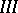, rings without non-zero finite idempotents. Every Baer ring decomposes in a unique way into a direct sum of rings of these types (see ).

Regular rings were introduced for the coordinization of continuous geometries, biregular rings in connection with the study of functional representations of rings, and Baer (and Rickart) rings in the study of rings of operators.

Non-associative regular rings have also been studied. See also-regular ring; Rickart ring.